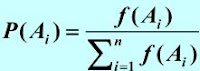## 2012年4月25日 星期三

### JAVA的HashMap對value排序

```import java.util.ArrayList;
import java.util.Collections;
import java.util.Comparator;
import java.util.HashMap;
import java.util.HashSet;
import java.util.Iterator;
import java.util.List;
import java.util.Map;
import java.util.Set;

public class neo {
//實作Comparator的介面宣告compare方法
private class ValueComparator implements Comparator<Map.Entry<String, Integer>>
{
public int compare(Map.Entry<String, Integer> mp1, Map.Entry<String, Integer> mp2)
{
//大到小mp2-mp1,小到大mp1-mp2
return mp2.getValue() - mp1.getValue();
}
}

public List<String> showsortedlist(Map<String, Integer> map)
{
//先把HashMap轉成List形式
List<Map.Entry<String, Integer>> sortedlist = new ArrayList<Map.Entry<String, Integer>>(map.size());
//把map中所有東西丟到sortedlist
ValueComparator vc = new ValueComparator();
//利用Collections.sort排序sortedlist
Collections.sort(sortedlist, vc);
final List<String> sortedvalue_keys = new ArrayList<String>(map.size());
Iterator iter = sortedlist.iterator();
while (iter.hasNext()) {
Entry<String, Integer> data = (Entry<String, Integer>) iter.next();
Object key = data.getKey();
Object val = data.getValue();
System.out.println(key+"=>"+val);
}
return sortedvalue_keys;
}
public static void main(String args[]){
Map<String,Integer> map = new HashMap<String,Integer>();
map.put("20030120" , new Integer (56));
map.put("20030118" , new Integer (19));
map.put("20030125" , new Integer (25));
map.put("20030122" , new Integer (32));
map.put("20030117" , new Integer (67));
map.put("20030123" , new Integer (34));
map.put("20030124" , new Integer (42));
map.put("20030121" , new Integer (19));
map.put("20030119" , new Integer (98));
Iterator iter = map.entrySet().iterator();
while (iter.hasNext()) {
Map.Entry entry = (Map.Entry) iter.next();
Object key = entry.getKey();
Object val = entry.getValue();
System.out.println(key+"=>"+val);
}
System.out.println("開始排序");
neo gosort  = new nothing();
gosort.showsortedlist(map);
}
}
```

## 2012年4月24日 星期二

### ubuntu安裝JDK&JRE

sudo apt-get install python-software-properties
sudo apt-get update
sudo apt-get install sun-java6-jdk

==================================================

sudo apt-get install python-software-properties
sudo apt-get update
sudo apt-get install sun-java6-jre sun-java6-plugin sun-java6-fonts

### Particle Filter tracking

Xk表示在時間k目標的位置Vk表示在時間k的系統誤差

Zk表示在時間k測量到的目標位置nk表示在時間k的測量誤差

Markov性質下

Chapman-Kolmogorov equation可化簡成下式

Prior Distribution : P(x0)  通常我們會假設為均勻分布
Transition Model : P(xt|xt-1)表示在兩個frame間目標物的移動情形
Observation Model : P(yt,xt) 表示在xt位置點上是否為目標物的likelihood

Rob Hess考慮了second-order的動態方程式

Observation Model p(yt|xt) 的部分他使用hsv histogram-base model

## 2012年4月23日 星期一

### differential convolution

```sigma = 1.5;
[x,y]=ndgrid(floor(-3*sigma):ceil(3*sigma),floor(-3*sigma):ceil(3*sigma));
%若sigma=2
%x=|-2 -2 -2 -2|
%  |-1 -1 -1 -1|
%  | 0  0  0  0|
%  | 1  1  1  1|
%  | 2  2  2  2|
DGaussx=-(x./(2*pi*sigma^4)).*exp(-(x.^2+y.^2)/(2*sigma^2));
DGaussy=-(y./(2*pi*sigma^4)).*exp(-(x.^2+y.^2)/(2*sigma^2));
Ix = imfilter(I,DGaussx,'conv');  %x方向梯度
Iy = imfilter(I,DGaussy,'conv');  %y方向梯度
```

```clear;
numLevels=3; %作幾層金字塔
window=9;    %小區域計算光流的區域呎吋
iterations=1;
alpha = 0.001;

hw = floor(window/2);
t0=clock;
pyramid1 = im1;
pyramid2 = im2;
for i=2:numLevels  %1最大3最小
im1 = impyramid(im1, 'reduce');
im2 = impyramid(im2, 'reduce');
pyramid1(1:size(im1,1), 1:size(im1,2), i) = im1;
pyramid2(1:size(im2,1), 1:size(im2,2), i) = im2;
end;
for p = 1:3
im1 = pyramid1(1:(size(pyramid1,1)/(2^(numLevels - p))), 1:(size(pyramid1,2)/(2^(numLevels - p))), (numLevels - p)+1);
im2 = pyramid2(1:(size(pyramid2,1)/(2^(numLevels - p))), 1:(size(pyramid2,2)/(2^(numLevels - p))), (numLevels - p)+1);
if p==1
u=zeros(size(im1));
v=zeros(size(im1));
else
%resizing
u = 2 * imresize(u,size(u)*2,'bilinear');
v = 2 * imresize(v,size(v)*2,'bilinear');
end
for r = 1:iterations
u=round(u);
v=round(v);
for i = 1+hw:size(im1,1)-hw
for j = 1+hw:size(im2,2)-hw
%作小區域的圖像patch1,patch2
patch1 = im1(i-hw:i+hw, j-hw:j+hw);

%moved patch
lr = i-hw+v(i,j);
hr = i+hw+v(i,j);
lc = j-hw+u(i,j);
hc = j+hw+u(i,j);

if (lr < 1)||(hr > size(im1,1))||(lc < 1)||(hc > size(im1,2))
%Regularized least square processing
else
patch2 = im2(lr:hr, lc:hc);

fx = conv2(patch1, 0.25* [-1 1; -1 1]) + conv2(patch2, 0.25*[-1 1; -1 1]);
fy = conv2(patch1, 0.25* [-1 -1; 1 1]) + conv2(patch2, 0.25*[-1 -1; 1 1]);
ft = conv2(patch1, 0.25*ones(2)) + conv2(patch2, -0.25*ones(2));

Fx = fx(2:window-1,2:window-1)';
Fy = fy(2:window-1,2:window-1)';
Ft = ft(2:window-1,2:window-1)';

A = [Fx(:) Fy(:)];
G=A'*A;

G(1,1)=G(1,1)+alpha; G(2,2)=G(2,2)+alpha;
%U = G反矩陣*A'*(-Ft)
U=1/(G(1,1)*G(2,2)-G(1,2)*G(2,1))*[G(2,2) -G(1,2);-G(2,1) G(1,1)]*A'*-Ft(:);
u(i,j)=u(i,j)+U(1); v(i,j)=v(i,j)+U(2);
end
end
end
end
end
x=135;
y=170;
figure(1)
imshow(im1);
hold on
plot(x,y,'r');
figure(2)
imshow(im1);
hold on
plot(x+u(x,y),y+v(x,y),'g');
u(x,y)
v(x,y)
```

## 2012年4月22日 星期日

### Genetic Algorithm solve Optimization Problem1)將問題要處理的所有資料編碼成稱為染色體(chromosonl)的字串
2)依問題的複雜程度，由所有染色體中隨機産生n個初始字串,這n個染色體可被稱為母體、族群(Population)
3)定義選擇規則(Selection),依據求解之條件設計的適應函數(fitness function)來選出)適應值高的染色體到交配池(mating  pool)中,挑選過程中依適應值高低比例複製(reproduction)到交配池中
4)再依交配(crossover)及突變 (mutation)過程的運算繁衍出子孫(offspring),到此為一個世代(Generation)
5)在毎一個世代中，都要對所新産生的染色體，評估其適應性(Fitness)評估否正在逼近問題的最佳解。
6)利用選擇規則(Selection)，將具有高適應性的子代保留，以形成新的母代族群進行下一輪的遺傳過程。

Selection (選擇)方法之一:crossover(交配)方法之一:
Single point crossover (單點交配)

Mutation (突變)方法之一:

1)執行設定的世代數
2)前後世代最佳值之差異低於設定的極小值
3)成熟率大於設定值

Step 1:

-------------------------------------------------

1                   14                            01110
2                   24                            11000
3                   17                            10001
4                      7                            00111
-------------------------------------------------

Step2:

-----------
x        f(x)
14      196
24      576
17      289
7         49
----------

Step3:

Step4:

Step5:

Step6：測試停止條件

### HNN(Hopfield Neural Network)

Wij= Σp Xip × Xjp

W13= Σp X1p × X3p =(+1)(+1) + (-1)(-1) +  (+1)(+1) + (-1)(-1) = 4

X = {+1, +1, +1, -1, +1, -1}

(第二次迭帶)：

可滿足穩定性的要求。

1)設定網路參數
Nout = 1 (一開始，輸出部份只有一個類別)
Nin = n (視每一組訓練範例的輸入變數之個數而定)

Wi1t = 1
Wi1b = 1/(1+Nin)

2)輸入一組訓練範例 X

3)計算每一個輸出層處理單元與所有輸入層處理單元間的匹配值net j
net j = Σi Wijb × Xi

net j* = max j(net j)

Vj* = (Σi Wij*t × Xi) /Σi Xi

4)測試警戒值 (由匹配值最大的輸出層節點開始)

5_1)修正該輸出層節點與所有輸入層節點的加權值(Wij*表示夠相似的該輸出神經元權值)
Wij*t = Wij*t × Xi
Wij*b = (Wij*t × Xi) / (0.5 + Σi(Wij*t × Xi ))

5_2)產生新的輸出神經元

Wi2t = Xi
Wi2b = Xi  / (0.5 + Σi(Xi))

6)若完成一個學習循環時沒有新增的類別(即5_2沒執行)則結束訓練

Wi1t = 1; Wt  = {1, 1, 1, 1, 1, 1}
Wi1b = 1/(1+Nin);  Wb  = {1/7, 1/7, 1/7, 1/7, 1/7, 1/7} = 1/7 {1, 1, 1, 1, 1, 1}

Wt _1 = {1, 0, 1, 0, 1, 0};
Wb _1= 1/(0.5+3){1, 0, 1, 0, 1, 0}

W t _2 = {0, 1, 0, 1, 0, 1};
W b _2 = 1/(0.5+3) {0, 1, 0, 1, 0, 1}

先看V1再看V2

Wt _1 = {1, 0, 1, 0, 0, 0};
Wb _1 = 1/(0.5+2) {1, 0, 1, 0, 0, 0}